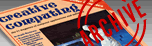`CREATIVE COMPUTING VOL. 9, NO. 12 / DECEMBER 1983 / PAGE 282`

 In the singular. (programming technique) David Leithauser. In the Singular One minor but annoying problem with computer output is the matter of singular and plural. For example, a game might have a program line which reads PRINT "YOU HAVE';N;"MISSILES LEFT'. This works fine if you have zero or more than one missile left, but it is grammatically incorrect if there is exactly one of the object (missiles in this case). Here I offer a simple technique for cleaning up the output. This technique relies on the fact that home computers actually treat logical comparisons (such as equal or greater than) as mathematical functions which generate a number. For example, if you tell the computer to PRINT 1=2, most computers will print a 0. If you tell the computer to PRINT 1=1, most computers will print either a 1 or a -1. TRS-80 Level II Basic, for example, gives a -1, while the Atari and Apple II give a 1. This fact can be used to choose a subscripted string variable, such as the letter S or an empty string. Taking the TRS-80 as an example, you can start the program with DIM S\$ (1): S\$ (0)="S'. The variable S\$ (1) is automatically assigned as an empty string. Then, replace a statement such as PRINT "YOU HAVE' N "MISSILES LEFT' with PRINT "YOU HAVE' N "MISSILES' S\$ (-(N=1)) "LEFT'. If N is not equal to 1 (say N=3), then N=1 generates a 0, and since S\$ (0);"S', the computer prints "YOU HAVE 3 MISSILES LEFT'. If N=1 the computer generates a -1, which becomes a positive 1 when the computer takes -(N=1). Since S\$ (1) is an empty string, the computer prints "YOU HAVE 1 MISSILES LEFT'. For computers in which a true statement generates a 1, such as the Apple II, the expression S\$ (-(N=1)) should be replaced by S\$ (N=1). If you do not know what number a true statement generates on your computer, just tell the computer to PRINT 1=1 and see what you get. For the Atari, which does not have subscripted strings, you can achieve the same result by using this technique to select a substring. Start your program with DIM S\$ (2):S "S'. Then use S\$ ((N=1) + 1, (N=1)+1) instead of S\$ (N=1). The technique can be extended to select the proper form for other words in the output. For example, if you have a statement such as PRINT "THREE ARE'; N; "ENEMY SHIPS LEFT' you can start your program (using the TRS-80 as an example again) with DIM S\$ (1), T\$ (1): S\$ (0)="S':T\$ (0)="ARE':T\$ (1)="IS' and change the output statement to read PRINT "THERE'; T\$ (-(N=1)); N; "ENEMY SHIP'; S\$ (-(N=1)); "LEFT'. On the Atari, this would be done by starting the program with DIM S\$ (2), T\$ (6):S "S':T "AREIS' and making the output statement PRINT "THREE' ;T\$ (3* (N=1)+1, 3* (N=1)+3); " '; N; "ENEMY SHIP' S\$ ((N=1) +1, (N=1)+1); "LEFT'.